Chapter 5 Class 12 Continuity and Differentiability

Class 12
Important Questions for exams Class 12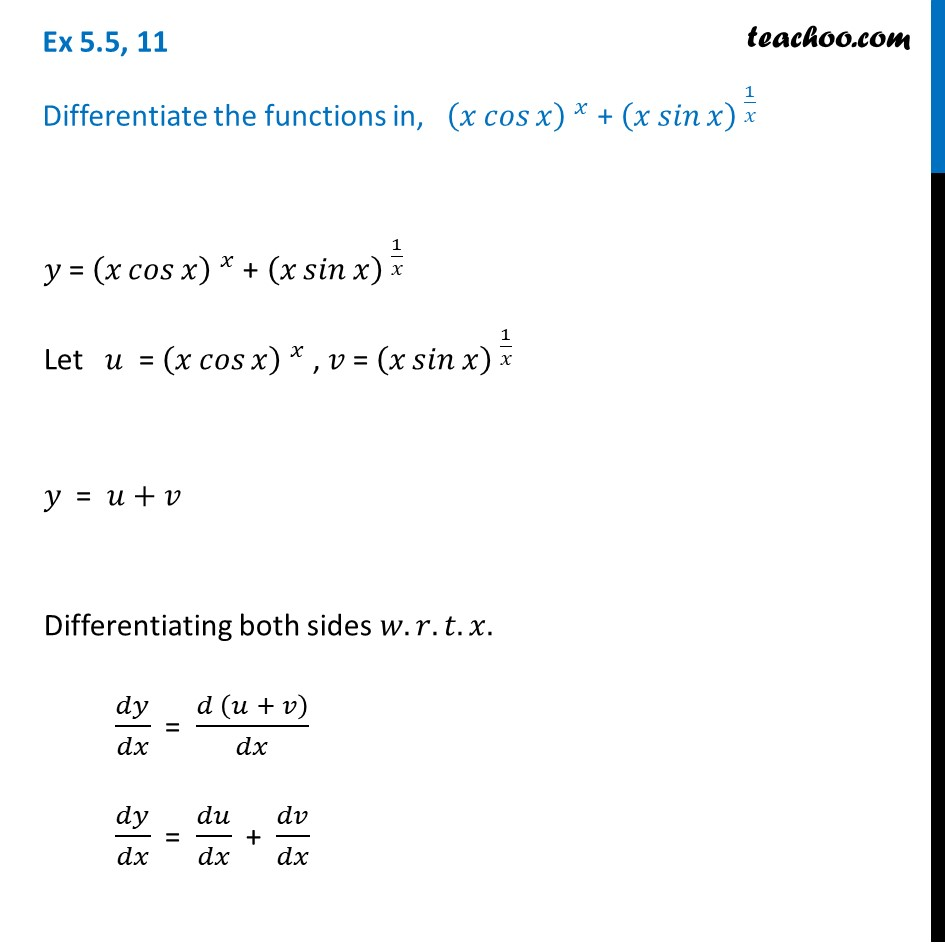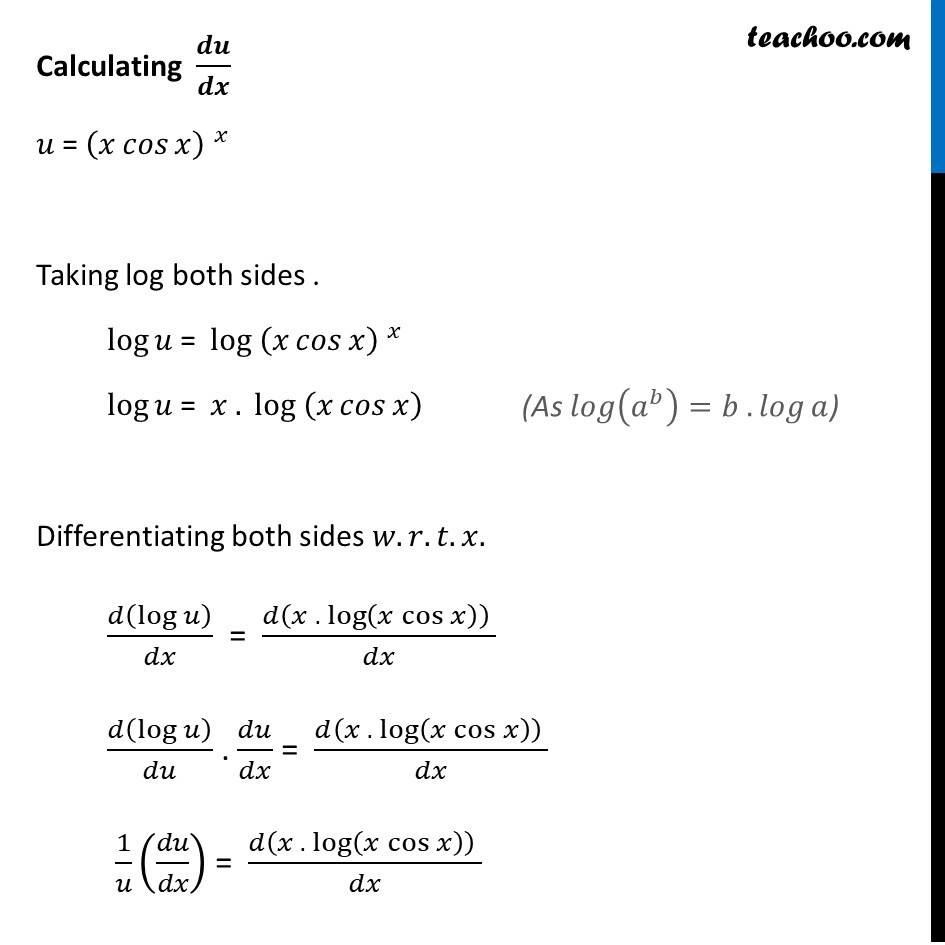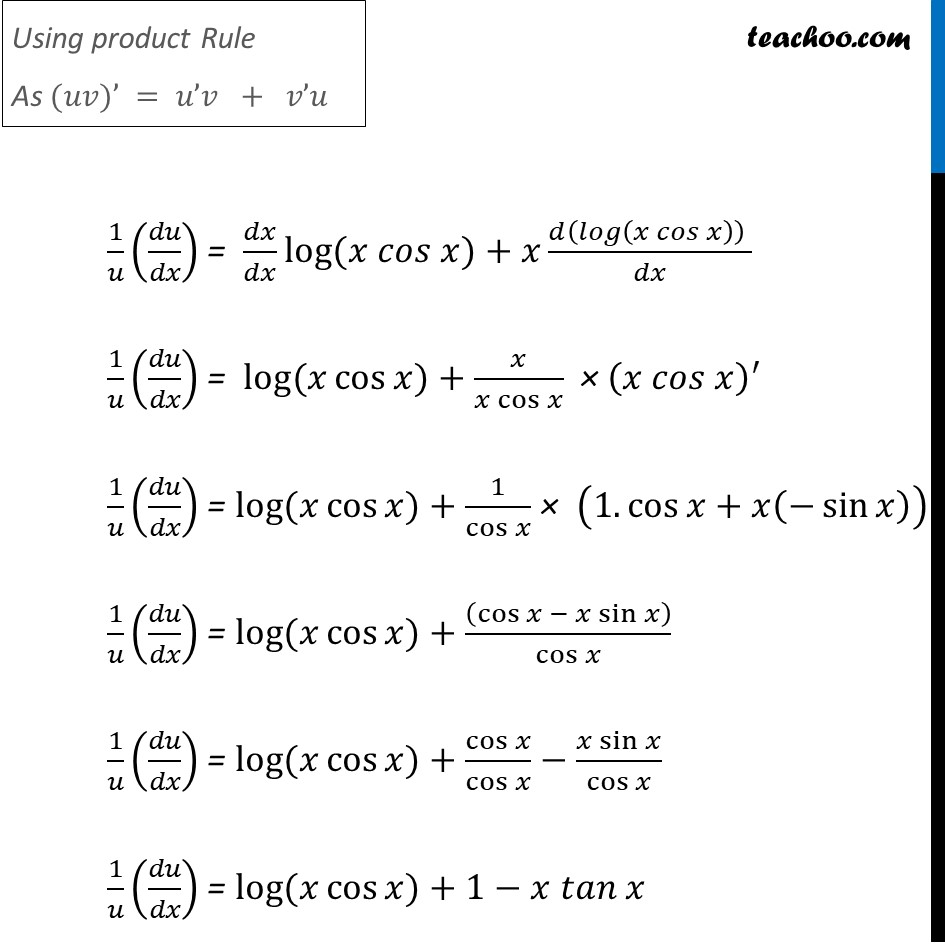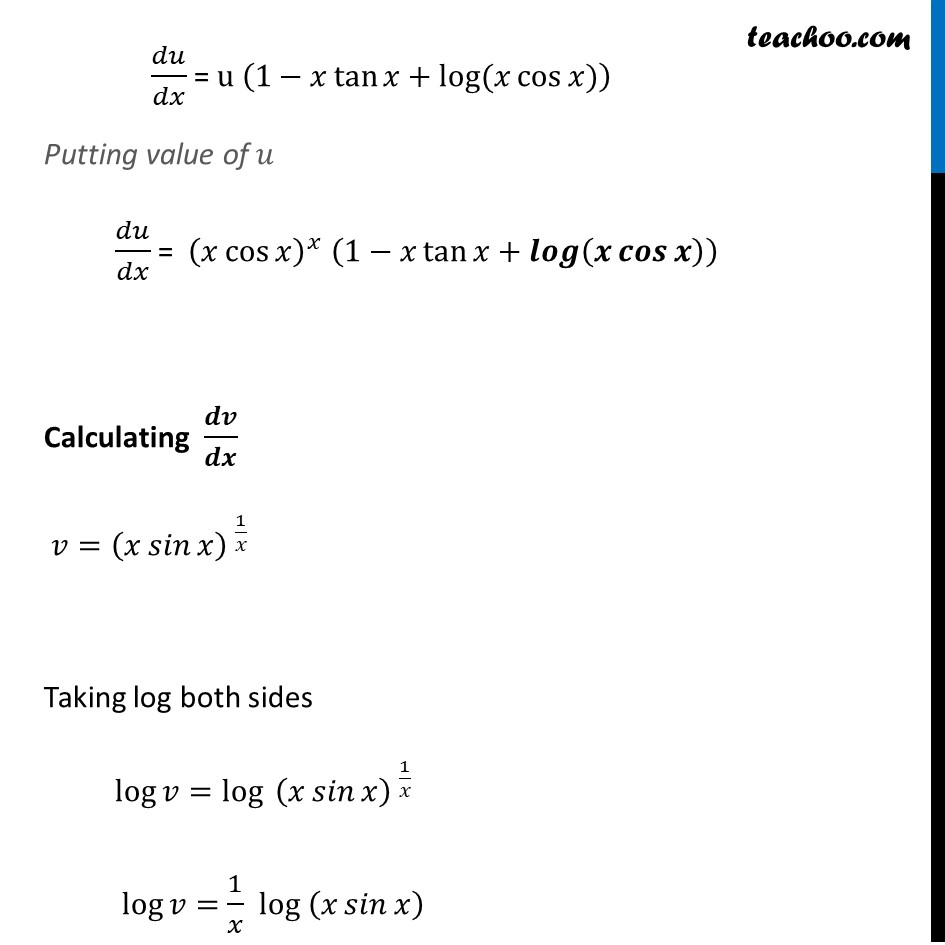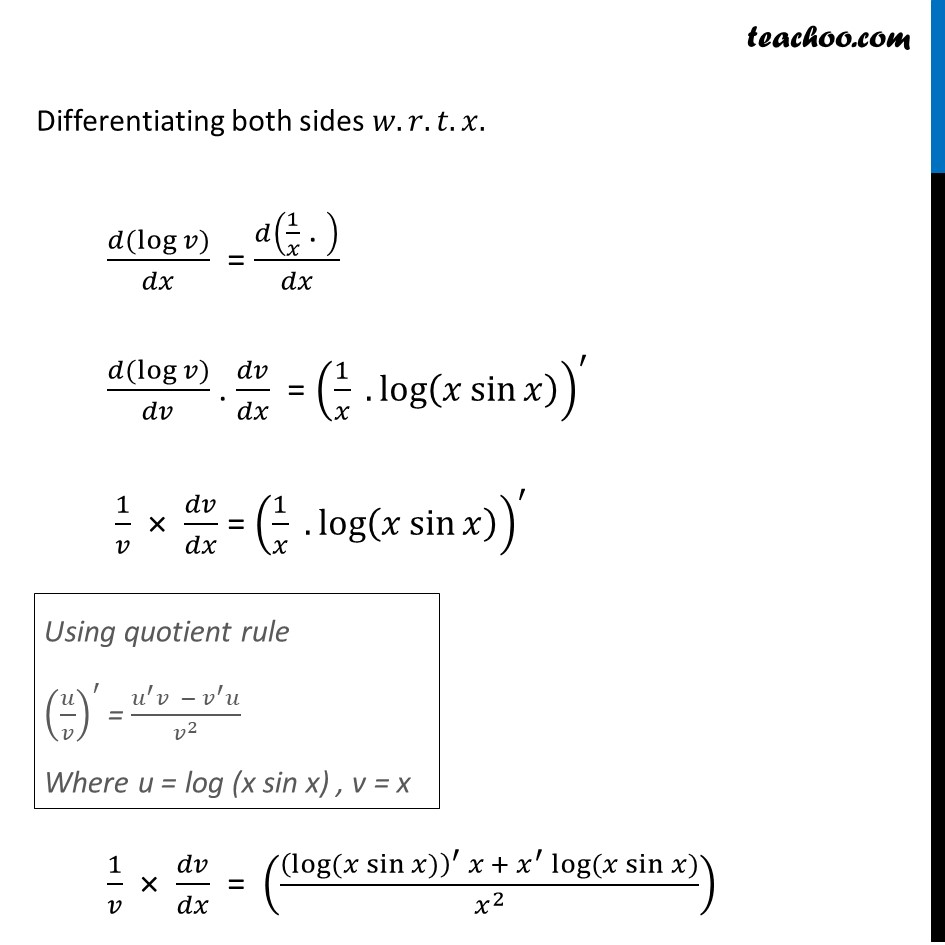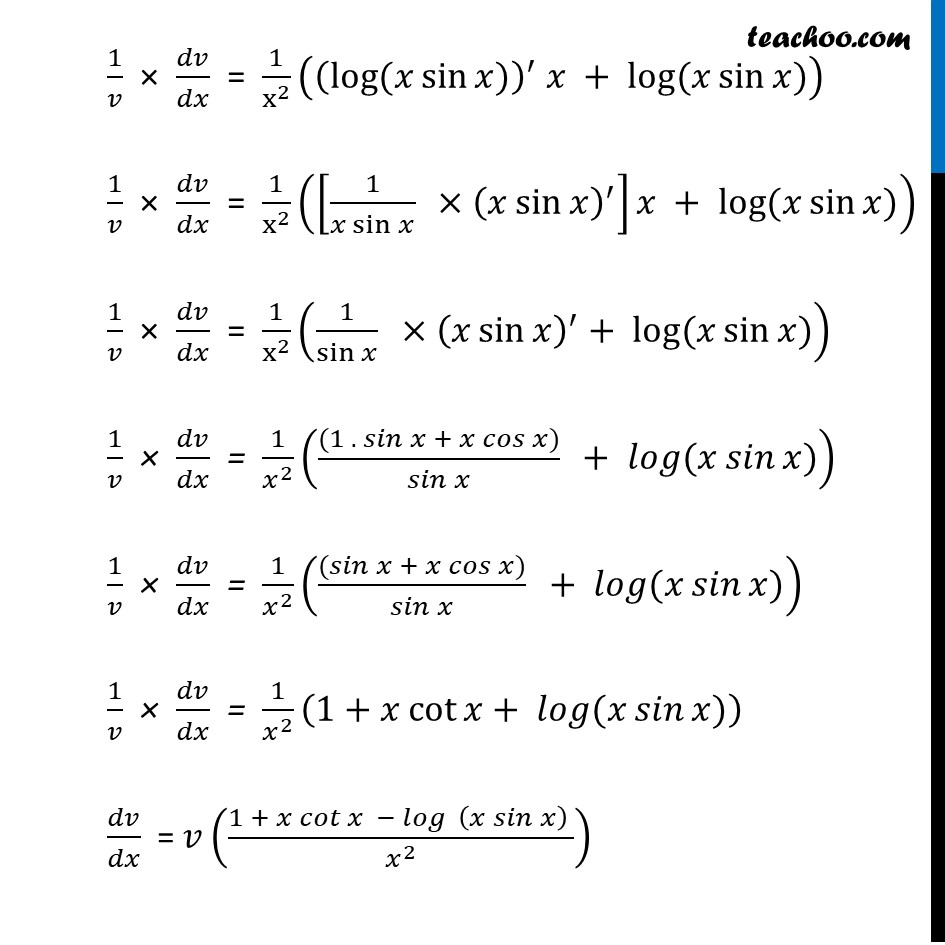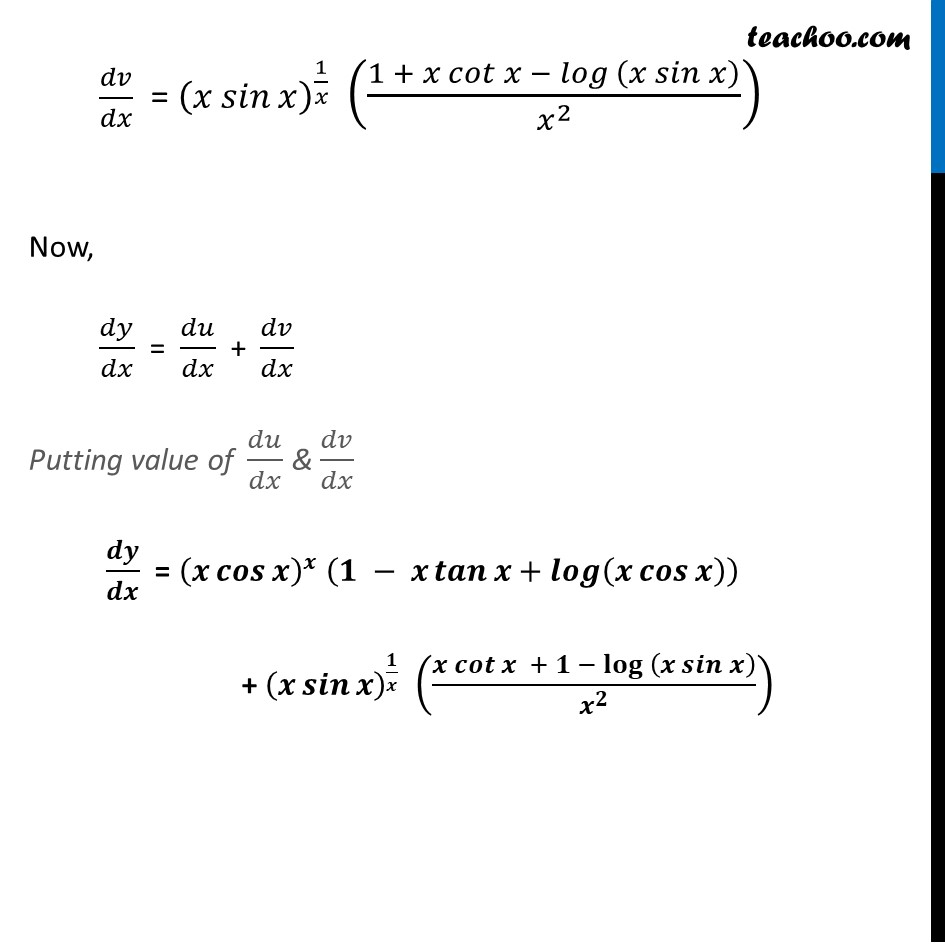Learn in your speed, with individual attention - Teachoo Maths 1-on-1 Class

### Transcript

Ex 5.5, 11 Differentiate the functions in, 〖(𝑥 𝑐𝑜𝑠⁡𝑥 ) 〗^𝑥 + 〖(𝑥 𝑠𝑖𝑛⁡𝑥 ) 〗^(1/𝑥)𝑦 = 〖(𝑥 𝑐𝑜𝑠⁡𝑥 ) 〗^𝑥 + 〖(𝑥 𝑠𝑖𝑛⁡𝑥 ) 〗^(1/𝑥) Let 𝑢 = 〖(𝑥 𝑐𝑜𝑠⁡𝑥 ) 〗^𝑥 , 𝑣 = 〖(𝑥 𝑠𝑖𝑛⁡𝑥 ) 〗^(1/𝑥) 𝑦 = 𝑢+𝑣 Differentiating both sides 𝑤.𝑟.𝑡.𝑥. 𝑑𝑦/𝑑𝑥 = (𝑑 (𝑢 + 𝑣))/𝑑𝑥 𝑑𝑦/𝑑𝑥 = 𝑑𝑢/𝑑𝑥 + 𝑑𝑣/𝑑𝑥 Calculating 𝒅𝒖/𝒅𝒙 𝑢 =〖 (𝑥 𝑐𝑜𝑠⁡𝑥 ) 〗^𝑥 Taking log both sides . log⁡𝑢 = log〖 (𝑥 𝑐𝑜𝑠⁡𝑥 ) 〗^𝑥 log⁡𝑢 = 𝑥 . log (𝑥 𝑐𝑜𝑠⁡𝑥 ) Differentiating both sides 𝑤.𝑟.𝑡.𝑥. 𝑑(log⁡𝑢 )/𝑑𝑥 = (𝑑(𝑥 . log⁡(𝑥 cos⁡𝑥 ) ) )/𝑑𝑥 𝑑(log⁡𝑢 )/𝑑𝑢 . 𝑑𝑢/𝑑𝑥 = (𝑑(𝑥 . log⁡(𝑥 cos⁡𝑥 ) ) )/𝑑𝑥 1/𝑢 (𝑑𝑢/𝑑𝑥) = (𝑑(𝑥 . log⁡(𝑥 cos⁡𝑥 ) ) )/𝑑𝑥 (As 𝑙𝑜𝑔⁡(𝑎^𝑏 )=𝑏 . 𝑙𝑜𝑔⁡𝑎) Using product Rule As (𝑢𝑣)’ = 𝑢’𝑣 + 𝑣’𝑢 1/𝑢 (𝑑𝑢/𝑑𝑥) = 𝑑𝑥/𝑑𝑥 log⁡〖(𝑥 𝑐𝑜𝑠 𝑥)〗+𝑥 (𝑑(𝑙𝑜𝑔⁡(𝑥 𝑐𝑜𝑠⁡𝑥 ) ) )/𝑑𝑥 1/𝑢 (𝑑𝑢/𝑑𝑥) = log⁡〖(𝑥 cos⁡𝑥)〗+𝑥/(𝑥 cos⁡𝑥 ) × (𝑥 𝑐𝑜𝑠 𝑥)^′ 1/𝑢 (𝑑𝑢/𝑑𝑥) = log⁡〖(𝑥 cos⁡𝑥)〗+1/cos⁡𝑥 × (1.cos⁡𝑥+𝑥(−sin⁡𝑥 )) 1/𝑢 (𝑑𝑢/𝑑𝑥) = log⁡〖(𝑥 cos⁡𝑥)〗+((cos⁡𝑥 − 𝑥 sin⁡𝑥 ))/cos⁡𝑥 1/𝑢 (𝑑𝑢/𝑑𝑥) = log⁡〖(𝑥 cos⁡𝑥)〗+cos⁡𝑥/cos⁡𝑥 −(𝑥 sin⁡𝑥)/cos⁡𝑥 1/𝑢 (𝑑𝑢/𝑑𝑥) = log⁡〖(𝑥 cos⁡𝑥)〗+1−𝑥 𝑡𝑎𝑛 𝑥 𝑑𝑢/𝑑𝑥 = u (1−𝑥 tan⁡𝑥+log⁡〖(𝑥 cos⁡𝑥 〗)) Putting value of 𝑢 𝑑𝑢/𝑑𝑥 = (𝑥 cos⁡𝑥 )^𝑥 (1−𝑥 tan⁡𝑥+𝒍𝒐𝒈⁡(𝒙 𝒄𝒐𝒔⁡𝒙 ) ) Calculating 𝒅𝒗/𝒅𝒙 𝑣=〖(𝑥 𝑠𝑖𝑛⁡𝑥 ) 〗^(1/𝑥) Taking log both sides log⁡𝑣=log⁡〖 〖(𝑥 𝑠𝑖𝑛⁡𝑥 ) 〗^(1/𝑥) 〗 log⁡𝑣= 1/𝑥 log (𝑥 𝑠𝑖𝑛⁡𝑥 ) Differentiating both sides 𝑤.𝑟.𝑡.𝑥. (𝑑(log⁡𝑣))/𝑑𝑥 = 𝑑(1/𝑥 " . " )/𝑑𝑥 (𝑑(log⁡𝑣))/𝑑𝑣 . 𝑑𝑣/𝑑𝑥 = (1/𝑥 .log⁡(𝑥 sin⁡𝑥 ) )^′ 1/𝑣 × 𝑑𝑣/𝑑𝑥 = (1/𝑥 .log⁡(𝑥 sin⁡𝑥 ) )^′ 1/𝑣 × 𝑑𝑣/𝑑𝑥 = (((log⁡〖(𝑥 sin⁡𝑥)〗 )^′ 𝑥 + 𝑥^′ log⁡〖(𝑥 sin⁡𝑥)〗)/𝑥^2 ) Using quotient rule (𝑢/𝑣)^′ = (𝑢^′ 𝑣 − 𝑣^′ 𝑢)/𝑣^2 Where u = log (x sin x) , v = x 1/𝑣 × 𝑑𝑣/𝑑𝑥 = 1/x^2 ((log⁡〖(𝑥 sin⁡𝑥)〗 )^′ 𝑥 + log⁡〖(𝑥 sin⁡𝑥)〗 ) 1/𝑣 × 𝑑𝑣/𝑑𝑥 = 1/x^2 ([1/(𝑥 sin⁡𝑥 ) \ ×(𝑥 sin⁡𝑥 )^′ ]𝑥 + log⁡〖(𝑥 sin⁡𝑥)〗 ) 1/𝑣 × 𝑑𝑣/𝑑𝑥 = 1/x^2 (1/sin⁡𝑥 \ ×(𝑥 sin⁡𝑥 )^′+ log⁡〖(𝑥 sin⁡𝑥)〗 ) 1/𝑣 × 𝑑𝑣/𝑑𝑥 = 1/𝑥^2 (((1 . 𝑠𝑖𝑛⁡𝑥 + 𝑥 𝑐𝑜𝑠⁡𝑥))/𝑠𝑖𝑛⁡𝑥 \ + 𝑙𝑜𝑔⁡〖(𝑥 𝑠𝑖𝑛⁡𝑥)〗 ) 1/𝑣 × 𝑑𝑣/𝑑𝑥 = 1/𝑥^2 (((𝑠𝑖𝑛⁡𝑥 + 𝑥 𝑐𝑜𝑠⁡𝑥))/𝑠𝑖𝑛⁡𝑥 \ + 𝑙𝑜𝑔⁡〖(𝑥 𝑠𝑖𝑛⁡𝑥)〗 ) 1/𝑣 × 𝑑𝑣/𝑑𝑥 = 1/𝑥^2 (1+𝑥 cot⁡𝑥+ 𝑙𝑜𝑔⁡〖(𝑥 𝑠𝑖𝑛⁡𝑥)〗 ) 𝑑𝑣/𝑑𝑥 = 𝑣((1 + 〖𝑥 𝑐𝑜𝑡〗⁡〖𝑥 〗 − 𝑙𝑜𝑔⁡〖 (𝑥 𝑠𝑖𝑛⁡𝑥 ) 〗)/𝑥^2 ) 𝑑𝑣/𝑑𝑥 = (𝑥 𝑠𝑖𝑛⁡𝑥 )^(1/𝑥) ((1 + 〖𝑥 𝑐𝑜𝑡〗⁡〖𝑥 〗− 𝑙𝑜𝑔 (𝑥 𝑠𝑖𝑛⁡𝑥 ))/𝑥^2 ) Now, 𝑑𝑦/𝑑𝑥 = 𝑑𝑢/𝑑𝑥 + 𝑑𝑣/𝑑𝑥 Putting value of 𝑑𝑢/𝑑𝑥 & 𝑑𝑣/𝑑𝑥 𝒅𝒚/𝒅𝒙 = (𝒙 𝒄𝒐𝒔⁡𝒙 )^𝒙 (𝟏 − 𝒙 𝒕𝒂𝒏⁡𝒙+𝒍𝒐𝒈⁡(𝒙 𝒄𝒐𝒔⁡𝒙 ) ) + (𝒙 𝒔𝒊𝒏⁡𝒙 )^(𝟏/𝒙) ((〖𝒙 𝒄𝒐𝒕〗⁡〖𝒙 〗 + 𝟏 − 𝐥𝐨𝐠 (𝒙 𝒔𝒊𝒏⁡𝒙 ))/𝒙^𝟐 )